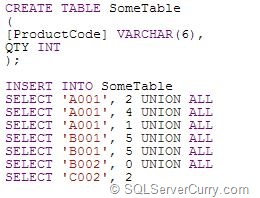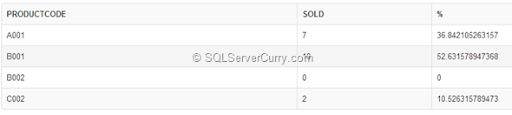### Using SUM OVER in SQL Server to Get % Sales

In some of our previous posts Aggregates without GroupBy SQL Server and SQL Server 2012 - Running total with SUM function‏, we have seen how to use SUM and OVER to calculate running totals and count without using the GroupBy clause.

Today we will use both the SUM and OVER function together to calculate Percentage(%) Sales
Consider the following table:To calculate what % of Qty sold each Product Code accounts for, use this code using SUM and OVER

SELECT [ProductCode], SUM(QTYSOLD) AS [Sold],
100.0 * SUM(QTYSOLD) / SUM(SUM(QTYSOLD)) OVER () AS [%]
FROM SomeTable
GROUP BY [ProductCode]

The code is pretty simple to understand. We are calculating the total items sold per Product and using a simple formula to calculate the % each Product Code has sold using SUM and OVER

OUTPUT